# Shapes

Shapes come in all forms and sizes. Have a look at the shapes below.

## Circle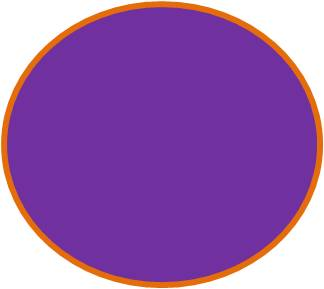## Diamond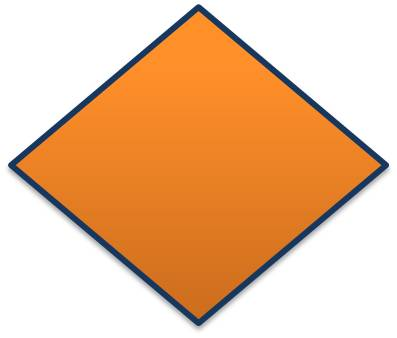## Rectangle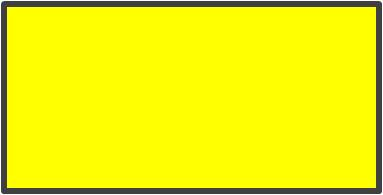## Cylinder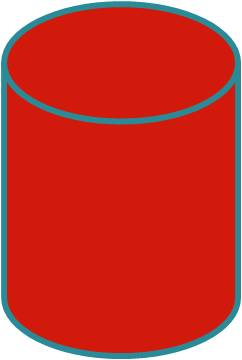## Hexagon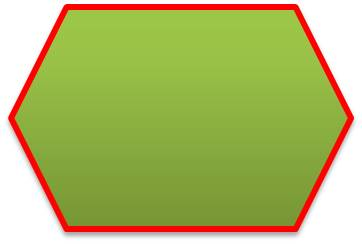## Oval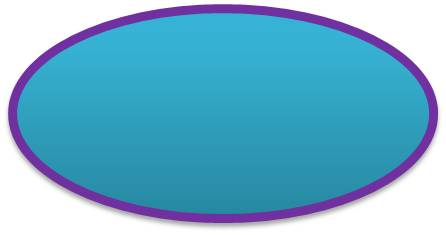## Traingle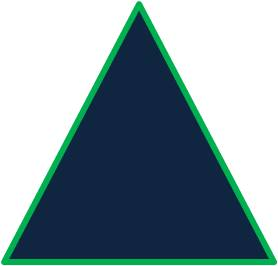## Square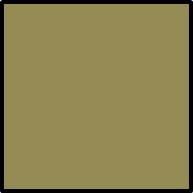# 2D Shapes

2D (dimensions) shapes are flat they do not have any volume.

The following are examples of 2D shapes:

• Triangle
• Rectangle
• Circle

# Triangles

Triangles have 3 sides and the total angles of a triangle add up to 180 degrees. There are different types of triangles.

# Isosceles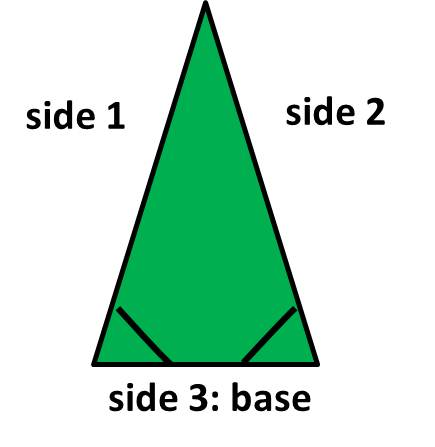The sides 1 and 2 are equal and the angles at the base are equal.

# Equilateral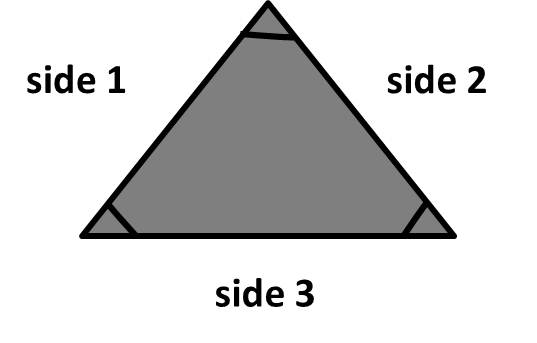The sides 1,2 and 3 are equal and all three angles are equal.

# Right-angled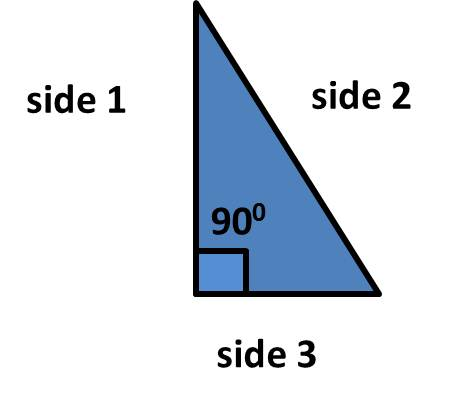A right angled triangle has a 90 degree angle.

## Square## Rectangle## Parallelogram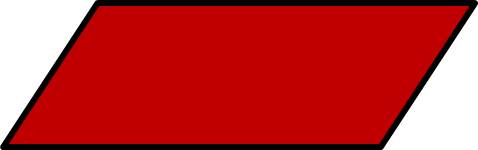# Area of a Triangle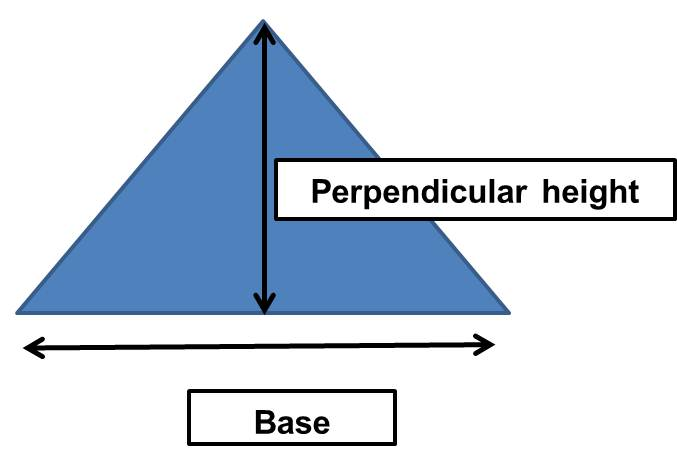Perpendicular means upright or vertical.

Area= ½ × base × perpendicular height
A=½ × b ×h

# Area of a Rectangle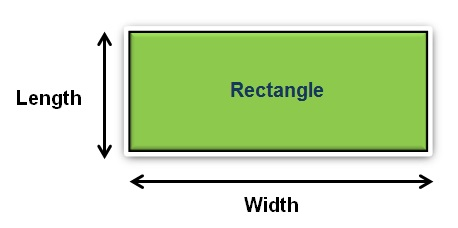Area= length × width
A= l × w

# Area of a Circle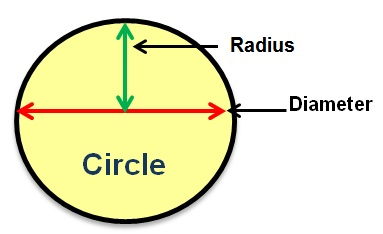A = π × r x r
or A = π × r²
The value for π is 3.14

# 3D Shapes

A 3D shape has width, depth and height.

## Cube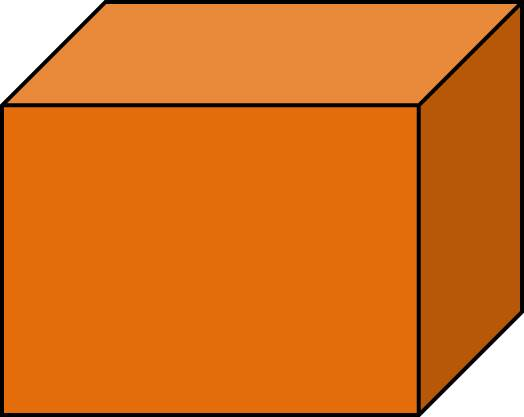## Cone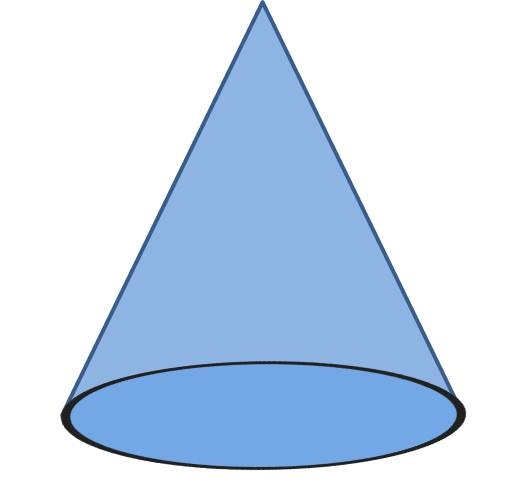## Cylinder## Volume of a Cylinder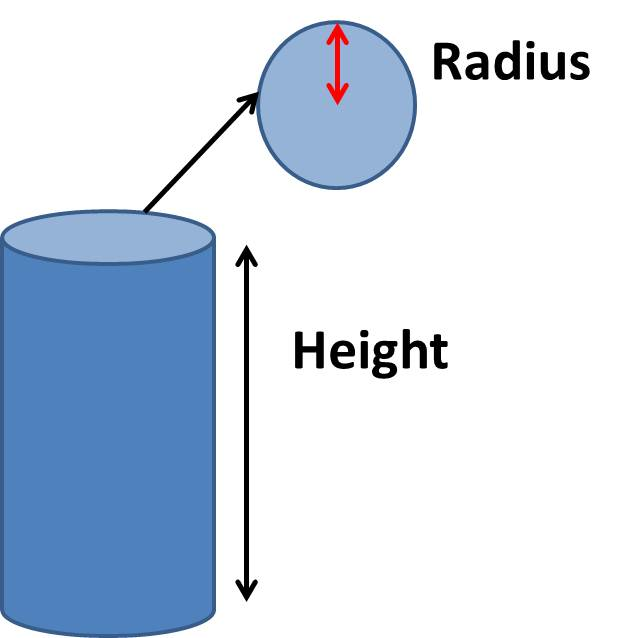V= πr²× h

## Volume of a Cuboid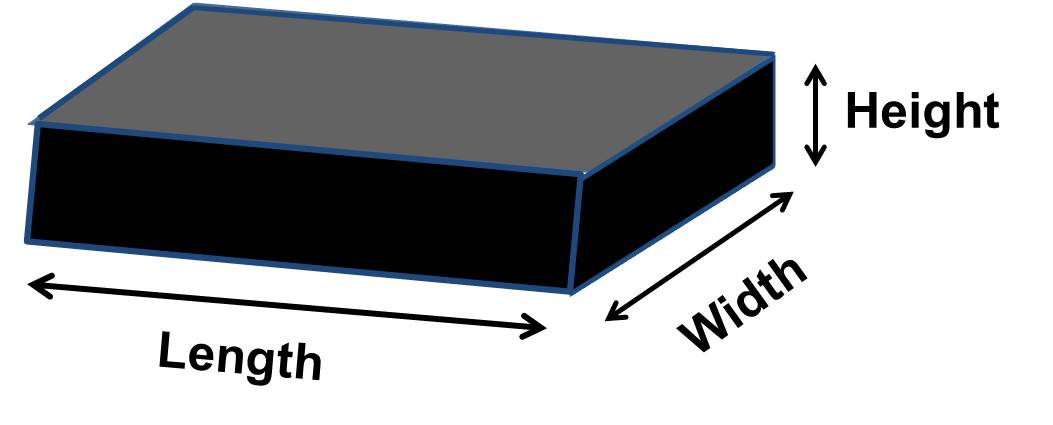Volume= length × width × height
V= l × w × h

Now try the Exercises!